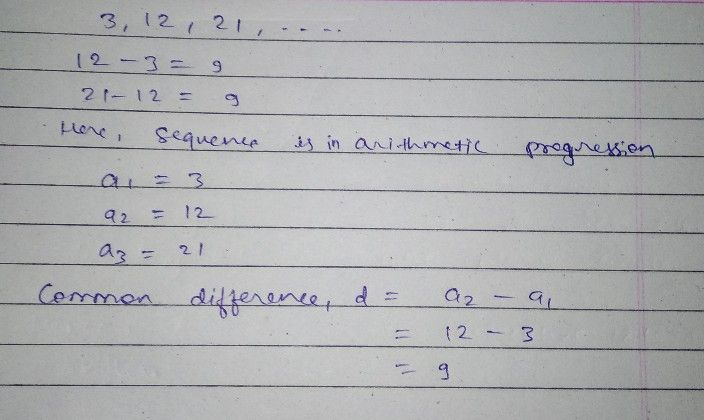Symbol
ProblemDetermine if the sequence below is arithmetic or geometric and determine the common difference $1$ ratio in simplest form. $△$ $3$ $12,21$ ...